# ML Aggarwal Solutions for Class 7 Maths Chapter 17 - Data Handling

ML Aggarwal Solutions for Class 7 Maths Chapter 17 – Data Handling are provided here to help students gain knowledge and have strong command over the subject, which will help secure high marks in their board exams. This chapter mainly deals with problems based on graphs. Students are provided with ML Aggarwal Solutions to help understand the concepts clearly from the exam point of view. Our solution module utilizes various shortcut tips and practical examples to explain all the questions in a simple and easily understandable language. The solutions are developed by the expert faculty team at BYJU’S, who have explained the concepts in detail, which is very helpful for students preparing for their board exams.

Chapter 17 – Data Handling provides solutions to questions related to each and every topic present in this chapter. Students can refer to ML Aggarwal Class 7 Solutions pdf and can easily download the readily available pdf for free, from the links given below and can start practising offline.

## Download the Pdf of ML Aggarwal Solutions for Class 7 Maths Chapter 17 – Data Handling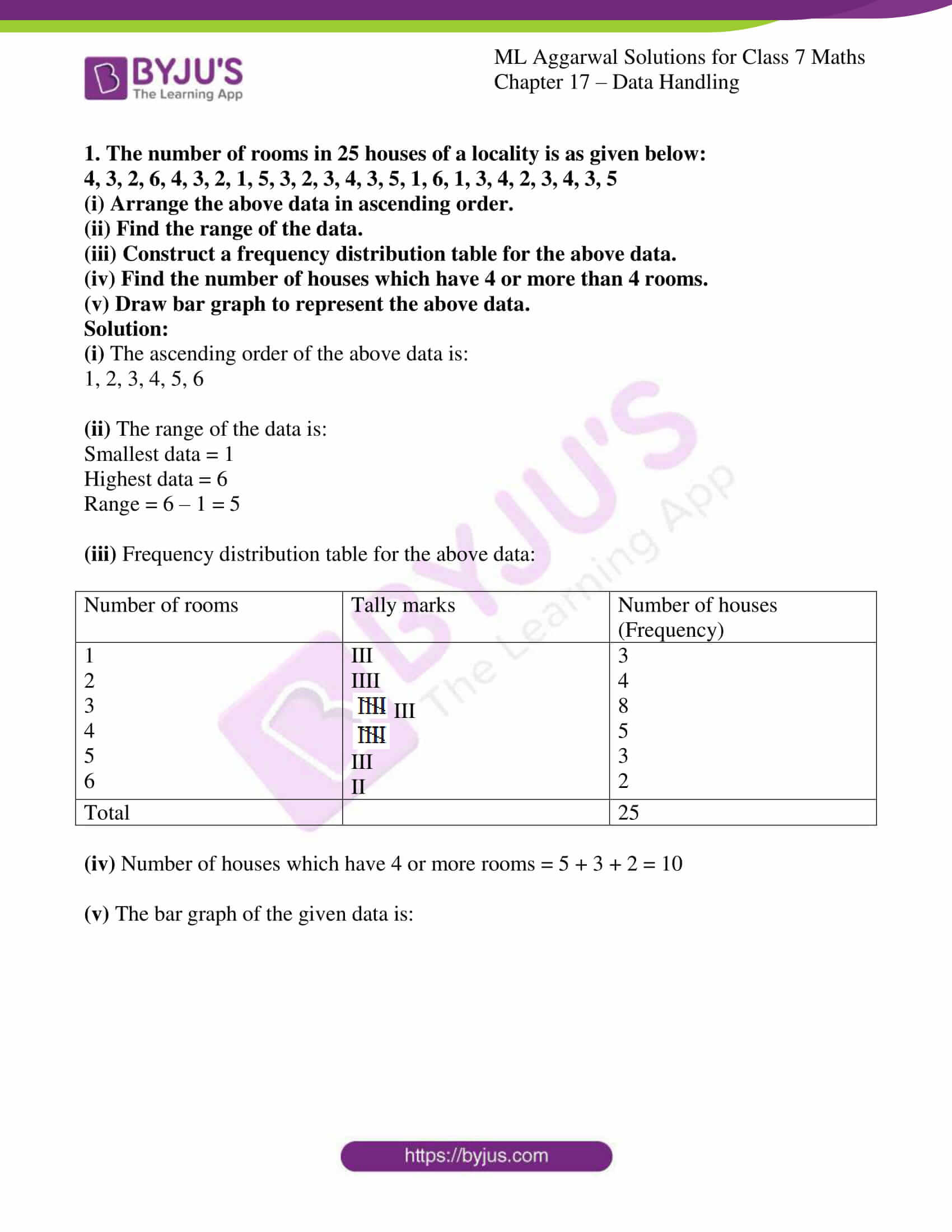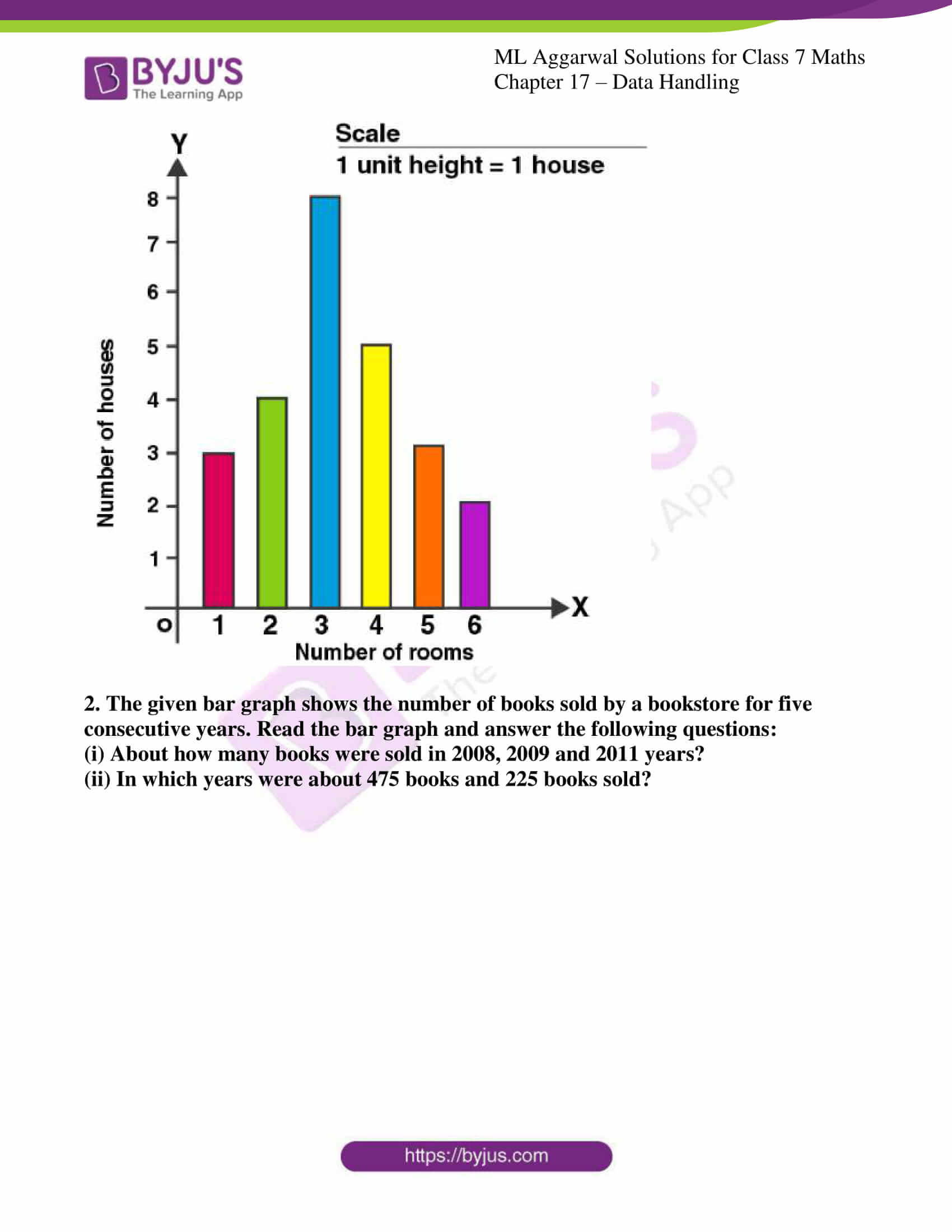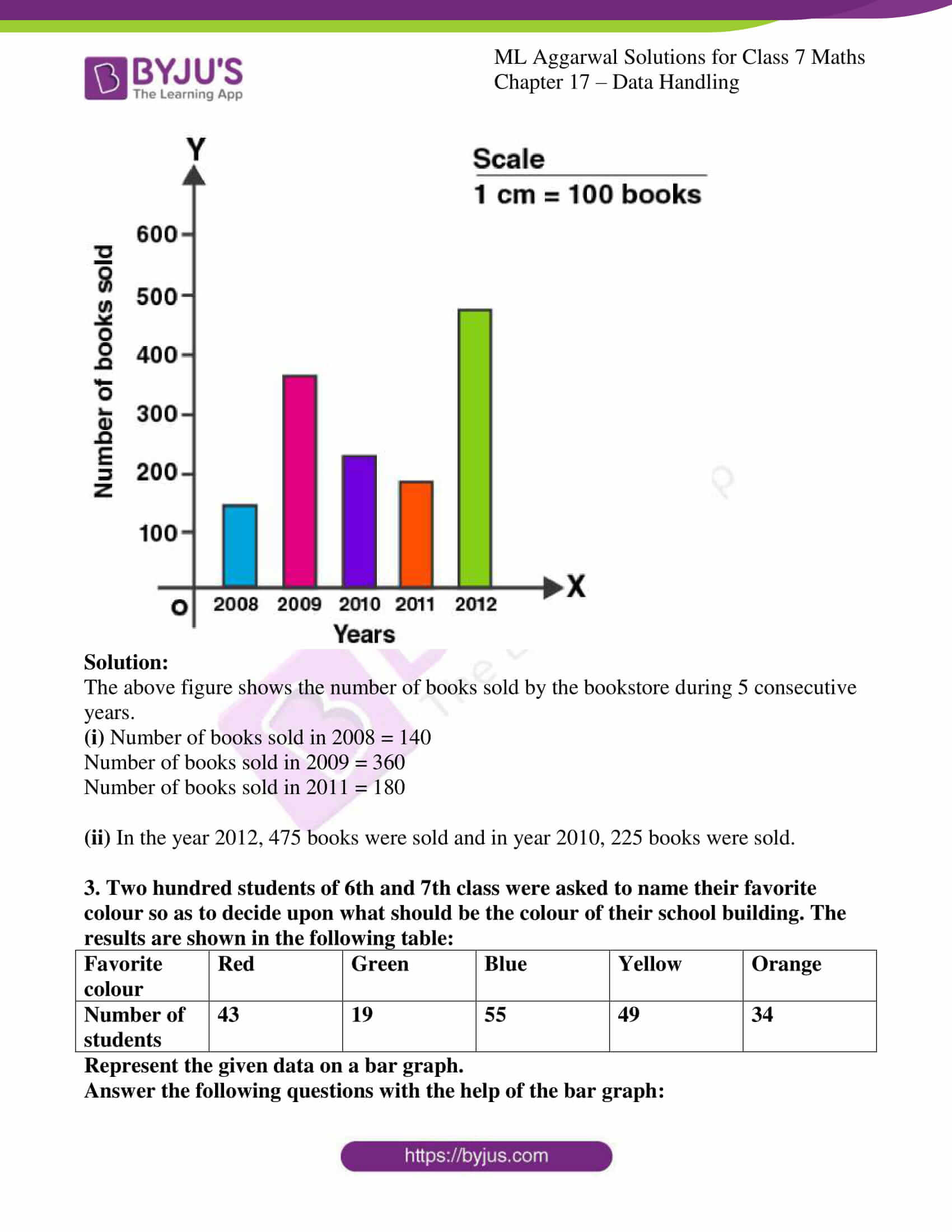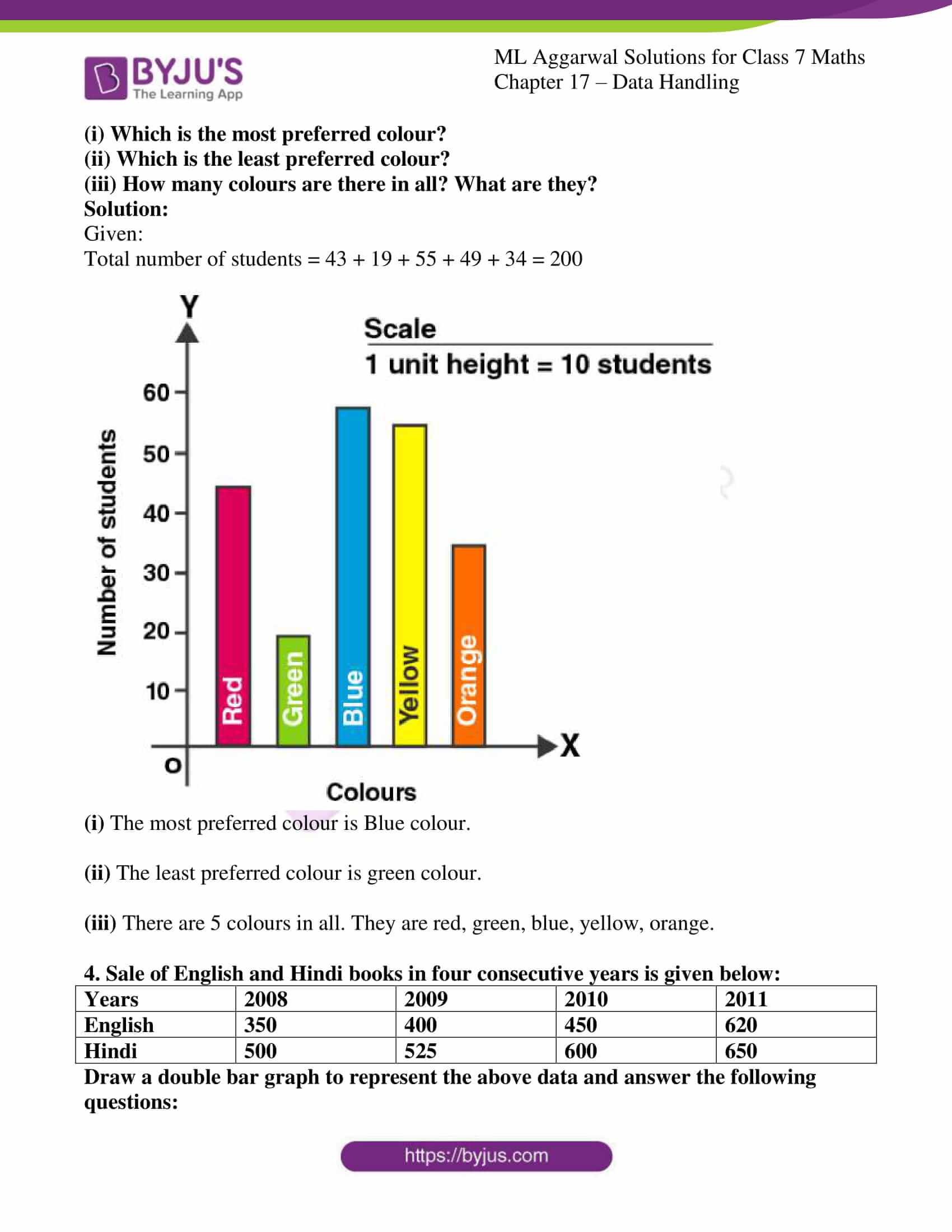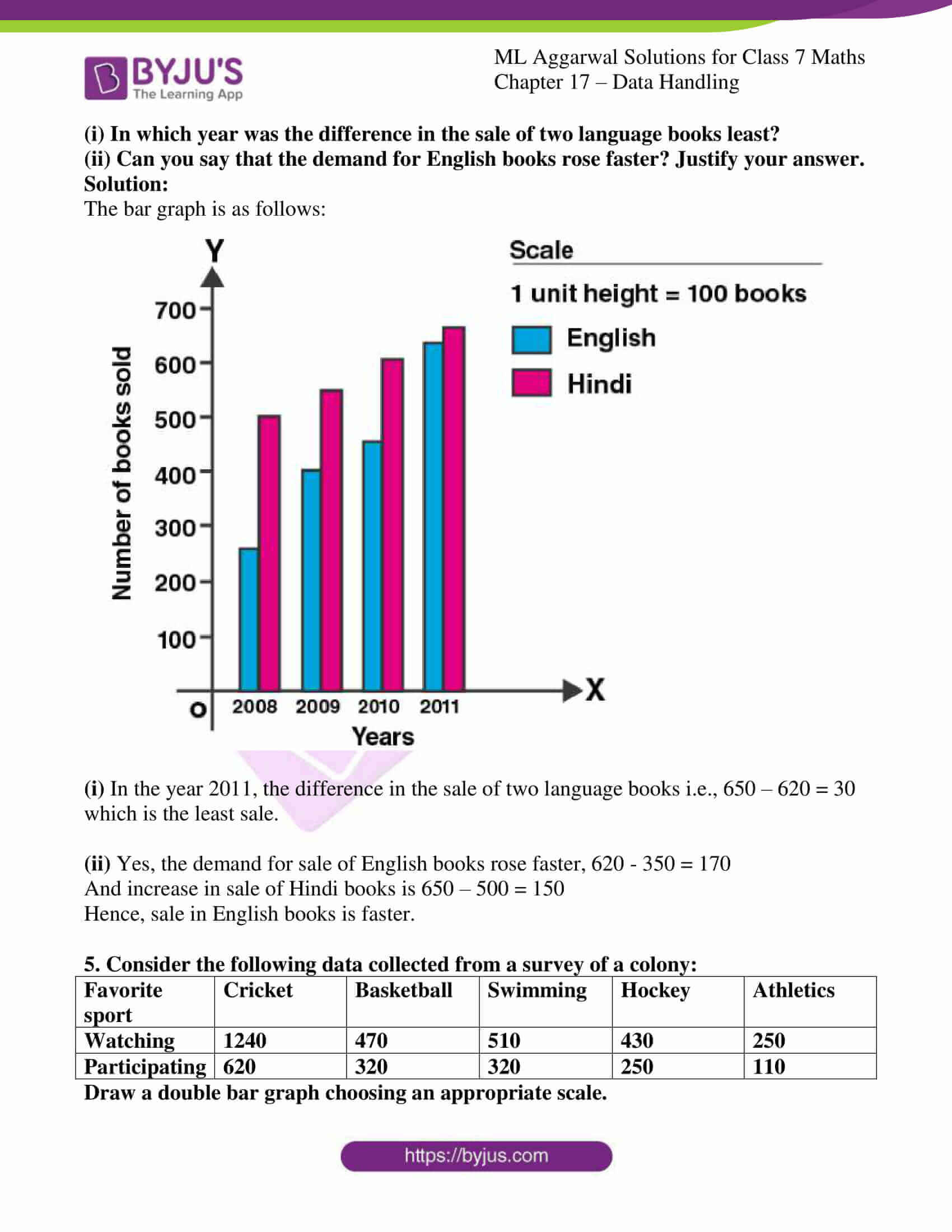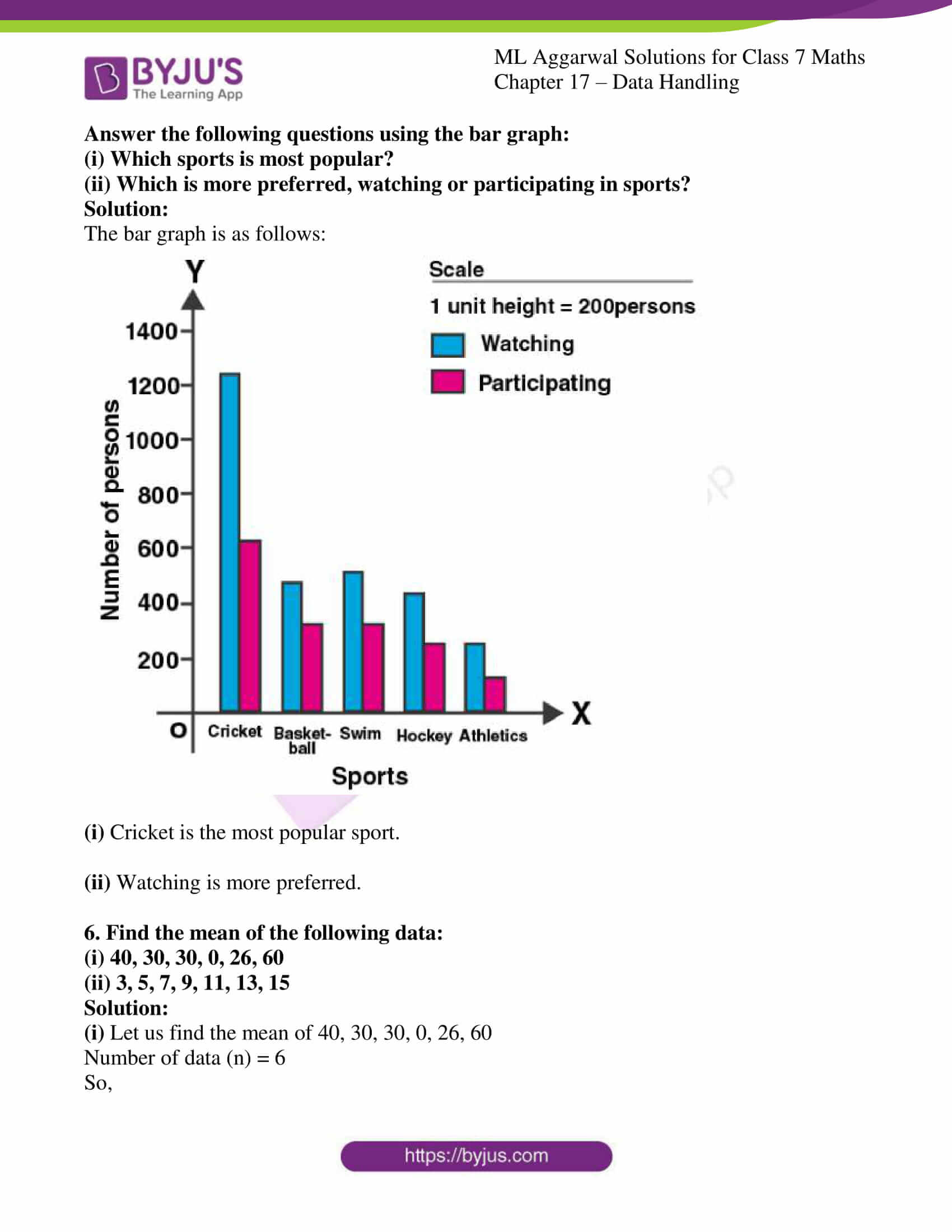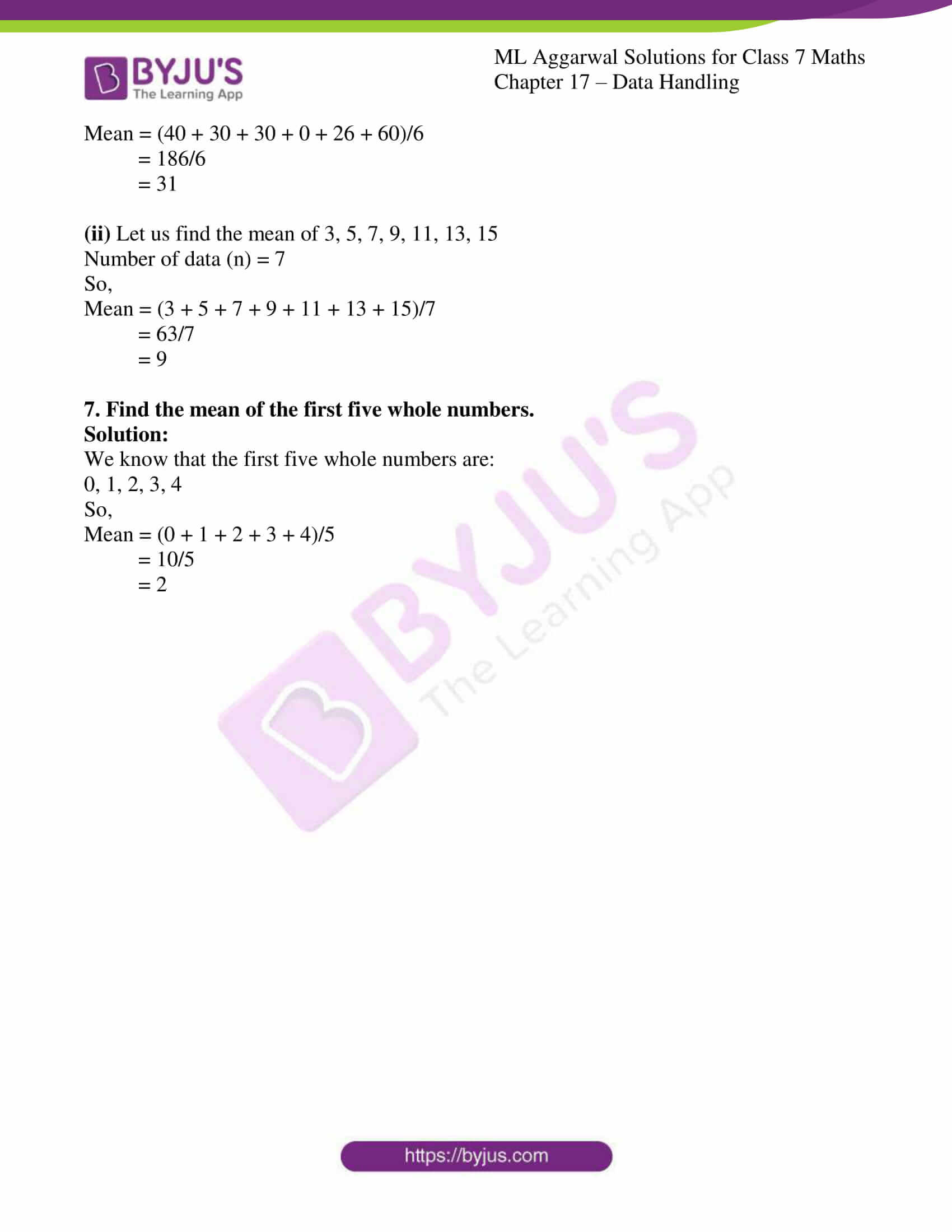### Access answers to ML Aggarwal Solutions for Class 7 Maths Chapter 17 – Data Handling

1. The number of rooms in 25 houses of a locality is as given below:
4, 3, 2, 6, 4, 3, 2, 1, 5, 3, 2, 3, 4, 3, 5, 1, 6, 1, 3, 4, 2, 3, 4, 3, 5
(i) Arrange the above data in ascending order.
(ii) Find the range of the data.
(iii) Construct a frequency distribution table for the above data.
(iv) Find the number of houses which have 4 or more than 4 rooms.
(v) Draw bar graph to represent the above data.
Solution:

(i) The ascending order of the above data is:

1, 2, 3, 4, 5, 6

(ii) The range of the data is:

Smallest data = 1

Highest data = 6

Range = 6 – 1 = 5

(iii) Frequency distribution table for the above data:

 Number of rooms Tally marks Number of houses (Frequency) 1 2 3 4 5 6 III IIII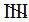III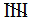III II 3 4 8 5 3 2 Total 25

(iv) Number of houses which have 4 or more rooms = 5 + 3 + 2 = 10

(v) The bar graph of the given data is: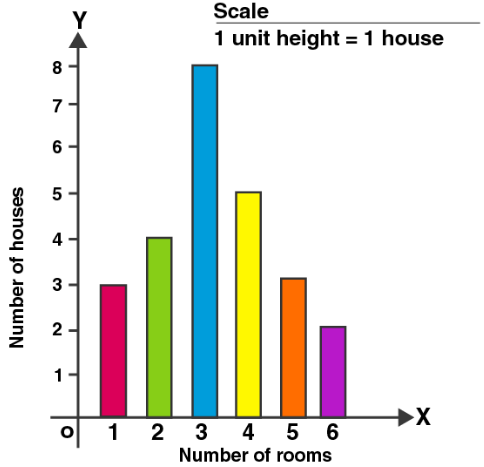2. The given bar graph shows the number of books sold by a bookstore for five consecutive years. Read the bar graph and answer the following questions:
(i) About how many books were sold in 2008, 2009 and 2011 years?
(ii) In which years were about 475 books and 225 books sold?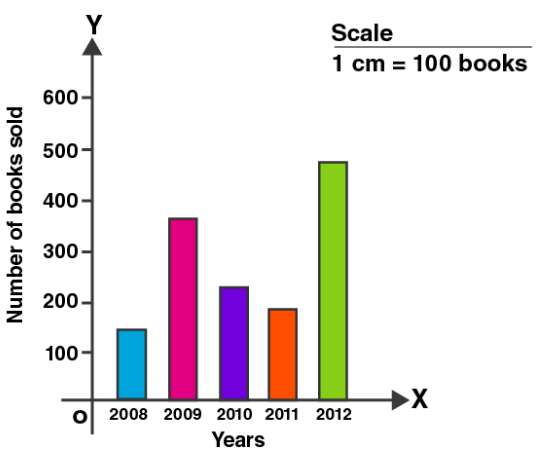Solution:

The above figure shows the number of books sold by the bookstore during 5 consecutive years.

(i) Number of books sold in 2008 = 140

Number of books sold in 2009 = 360

Number of books sold in 2011 = 180

(ii) In the year 2012, 475 books were sold and in year 2010, 225 books were sold.

3. Two hundred students of 6th and 7th class were asked to name their favorite colour so as to decide upon what should be the colour of their school building. The results are shown in the following table:

 Favorite colour Red Green Blue Yellow Orange Number of students 43 19 55 49 34

Represent the given data on a bar graph.
Answer the following questions with the help of the bar graph:
(i) Which is the most preferred colour?
(ii) Which is the least preferred colour?
(iii) How many colours are there in all? What are they?
Solution:

Given:

Total number of students = 43 + 19 + 55 + 49 + 34 = 200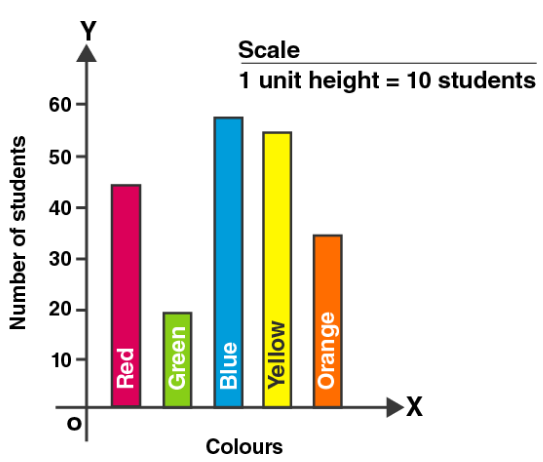(i) The most preferred colour is Blue colour.

(ii) The least preferred colour is green colour.

(iii) There are 5 colours in all. They are red, green, blue, yellow, orange.

4. Sale of English and Hindi books in four consecutive years is given below:

 Years 2008 2009 2010 2011 English 350 400 450 620 Hindi 500 525 600 650

Draw a double bar graph to represent the above data and answer the following questions:
(i) In which year was the difference in the sale of two language books least?
(ii) Can you say that the demand for English books rose faster? Justify your answer.
Solution:

The bar graph is as follows: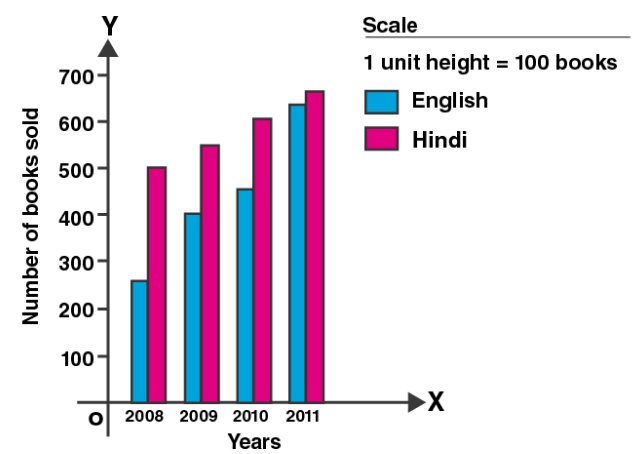(i) In the year 2011, the difference in the sale of two language books i.e., 650 – 620 = 30 which is the least sale.

(ii) Yes, the demand for sale of English books rose faster, 620 – 350 = 170

And increase in sale of Hindi books is 650 – 500 = 150

Hence, sale in English books is faster.

5. Consider the following data collected from a survey of a colony:

 Favorite sport Cricket Basketball Swimming Hockey Athletics Watching 1240 470 510 430 250 Participating 620 320 320 250 110

Draw a double bar graph choosing an appropriate scale.
Answer the following questions using the bar graph:
(i) Which sports is most popular?
(ii) Which is more preferred, watching or participating in sports?
Solution:

The bar graph is as follows: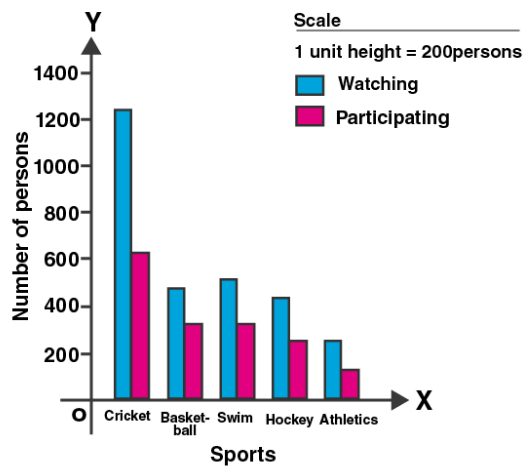(i) Cricket is the most popular sport.

(ii) Watching is more preferred.

6. Find the mean of the following data:
(i) 40, 30, 30, 0, 26, 60
(ii) 3, 5, 7, 9, 11, 13, 15
Solution:

(i) Let us find the mean of 40, 30, 30, 0, 26, 60

Number of data (n) = 6

So,

Mean = (40 + 30 + 30 + 0 + 26 + 60)/6

= 186/6

= 31

(ii) Let us find the mean of 3, 5, 7, 9, 11, 13, 15

Number of data (n) = 7

So,

Mean = (3 + 5 + 7 + 9 + 11 + 13 + 15)/7

= 63/7

= 9

7. Find the mean of the first five whole numbers.
Solution:

We know that the first five whole numbers are:

0, 1, 2, 3, 4

So,

Mean = (0 + 1 + 2 + 3 + 4)/5

= 10/5

= 2